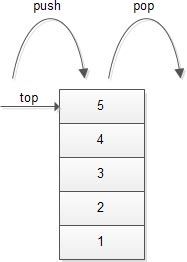# C Stack Using Array

Summary: in this tutorial, you will learn about stack data structure and how to implement a C stack using an array.

##Introduction to stack data structure

A stack is a data structure that works based on the principle of last-in-first-out (LIFO). It means the last element that was added to the stack must be the first one to be removed.

Stack data structure has many applications such as parsing the syntax of expressions, managing run-time memory (used in Java virtual machine) and using in searching algorithms.

## C stack operations

There are two main operations that you can perform in the stack:

• `push`: allows you to insert an element into the stack.
• `pop`: allows you to remove an element from the stack.

In addition, a stack can have:

• `empty`: checks if the stack is empty
• `full`: checks if the stack is full
• `init`: initialize the stack pointer
• `display`: displays the content of the stack

## C stack implementation using array

The following is C source code that demonstrates a stack data structure.

Stack header file:  `stack.h`

Stack code file: `stack.c` (stack implementation in C)

We can test the stack data structure that uses an array in the main.c file (stack program in C)

The following is the output of the program:

You can download the C stack code implemented using an array via the following link:

C Stack (2894 downloads)

In this tutorial, you have learned what a stack is and how to implement C stack data structure using an array.

• Was this tutorial helpful ?
• YesNo